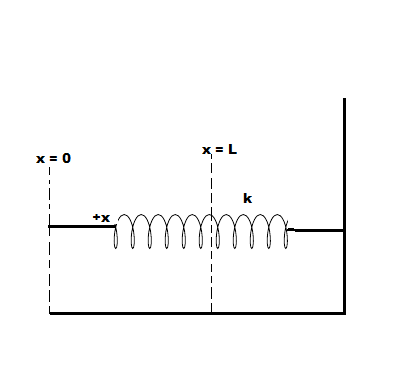# Consider a spring, with spring constant k, one end of which is attached to a wall.The spring is...

## Question:

Consider a spring, with spring constant k, one end of which is attached to a wall. Initially the spring is not stretched, with the unconstrained end of the spring at position {eq}x = 0 {/eq}.

The spring is now compressed so that the unconstrained end moves from {eq}x = 0 {/eq} to {eq}x = L {/eq}.

Using the work integral {eq}\displaystyle W = \int\limits_{x_i}^{x_f} \vec F (\vec x) \cdot \vec{ dx} {/eq}, find the work done by the spring as it is compressed. Express the work done by the spring in terms of k and L.## Work required to Stretch the Spring:

Using integration, we can calculate the work required to stretch the spring. The spring force is the resisting force of the spring against its distortion, and it is growing linearly with the extension or contraction of the spring. The work required to create a double deformation is four times the work required to create an initial deformation.

## Answer and Explanation: 1

Become a Study.com member to unlock this answer! Create your account

Given data:

• {eq}k {/eq} is the spring constant
• {eq}W {/eq} is the work is done by spring
• {eq}F {/eq} is the spring force

Using Hooke's law:

{...

See full answer below.

#### Learn more about this topic:Hooke's Law & the Spring Constant: Definition & Equation

from

Chapter 4 / Lesson 19
202K

After watching this video, you will be able to explain what Hooke's Law is and use the equation for Hooke's Law to solve problems. A short quiz will follow.